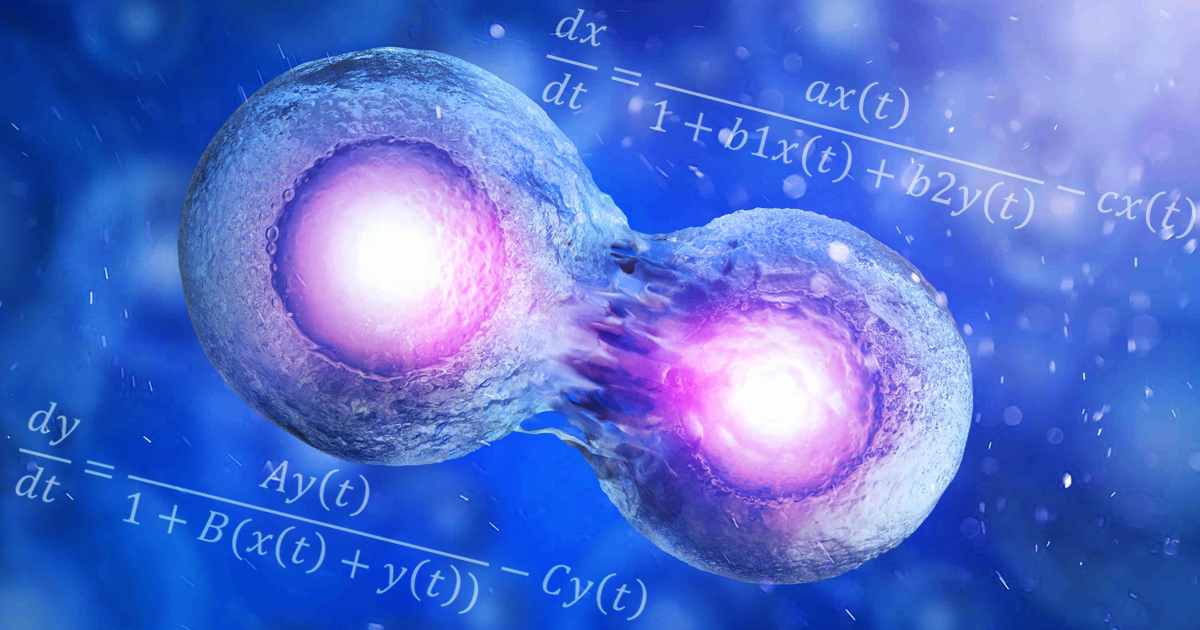# Ph.D. thesis in Biomathematics ranked in the top three best doctoral theses of the 2019 Reinhart-Heinrich Award

Jan 20, 2021The Reinhart-Heinrich Doctoral Thesis Award Committee from the European Society for Mathematical and Theoretical Biology (ESMTB) has classified the Ph.D. thesis “Analysis of Some Mathematical Models of Cell Dynamics in Hematology” in the top three best doctoral theses of 2019 in the area of Mathematical and Theoretical Biology.

The thesis deals with the analysis of some mathematical models of cell dynamics and mathematical optimization problems of drug delivery applied to one type of cancer of the white blood cells (chronic myeloid leukemia). In his work, the author takes into consideration basic concepts, methods and results from the theory of differential equations, such as: existence, uniqueness, boundedness, continuous dependence on data and stability of solutions. Apart from pure analytical work numerical simulations are also considered to extend the theoretical results.

The thesis “Analysis of Some Mathematical Models of Cell Dynamics in Hematology” is structured in four chapters. Chapter 1 is entirely dedicated to a medical background used throughout this work.  Chapter 2 illustrates a two-dimensional mathematical model of cell dynamics which shows us the transition process from the normal formation of cellular components to the chronic and accelerated-acute stages in myeloid leukemia. The purpose of Chapter 3 is to develop a mathematical approach of optimal therapy for individual patients in chronic myeloid leukemia which more precisely is considered a mathematical optimization problem based on the two-dimensional mathematical model introduced in Chapter 2 and are being analyzed different scenarios of the treatment. Chapter 4 is all about the study of a mathematical model of stem cell dynamics after bone marrow transplantation in this type of leukemia. At the end of the thesis, Conclusions and Further Research is presented, along with an Appendix that contains the source code of the numerical simulations.

The thesis is based on seven scientific papers already published in prestigious journals and/or publishing houses.

On the author:

Dr. Lorand Gabriel Parajdi was between 2015 and 2019, a Ph.D. student in the Doctoral School of the Faculty of Mathematics and Computer Science. The Ph.D. thesis was elaborated under the supervision of Prof. Dr. Radu Precup and in collaboration with researchers and medical doctors from the Department of Hematology, “Ion Chiricuță” Clinical Cancer Center, Cluj-Napoca, Romania. Lorand Gabriel Parajdi defended his Ph.D. thesis at Babeș-Bolyai University in 2019. In 2020 he became an Assistant Professor in the Department of Mathematics, Faculty of Mathematics and Computer Science. His fields of interest are: Ordinary Differential Equations, Partial Differential Equations, Mathematical Modelling in Medicine and Biology and Nonlinear Dynamics.

For further information on Dr. Lorand Gabriel Parajdi’s works, please refer to his personal webpage: cs.ubbcluj.ro/~lorand/

His Ph.D. Thesis Summary can be consulted following the link below: